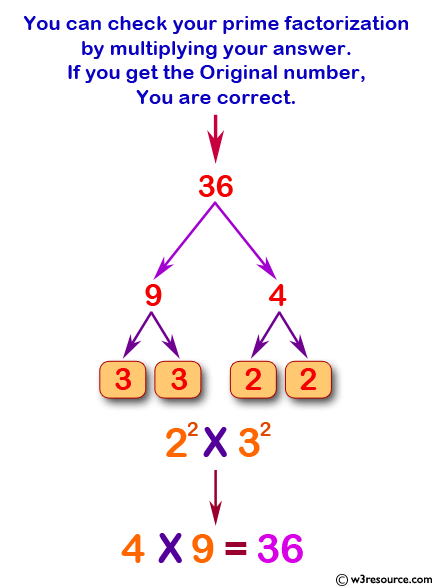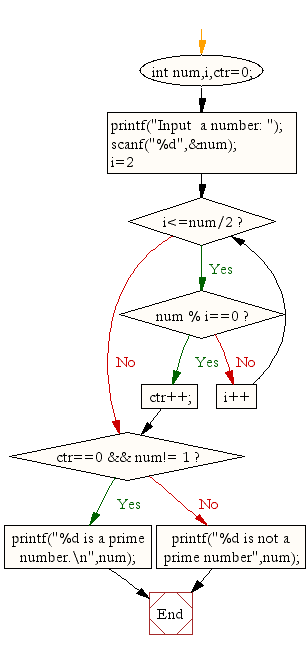﻿ C Program: Check whether a given number is prime or not - w3resource# C Exercises: Check whether a given number is prime or not

## C For Loop: Exercise-32 with Solution

Write a C program to determine whether a given number is prime or not.

Pictorial Presentation:Sample Solution:

C Code:

``````#include <stdio.h>

void main(){

int num,i,ctr=0;
printf("Input  a number: ");
scanf("%d",&num);
for(i=2;i<=num/2;i++){
if(num % i==0){
ctr++;
break;
}
}
if(ctr==0 && num!= 1)
printf("%d is a prime number.\n",num);
else
printf("%d is not a prime number",num);
}
```
```

Sample Output:

```Input  a number: 13
13 is a prime number.
```

Flowchart:C Programming Code Editor:

Improve this sample solution and post your code through Disqus.

What is the difficulty level of this exercise?

Test your Programming skills with w3resource's quiz.

﻿